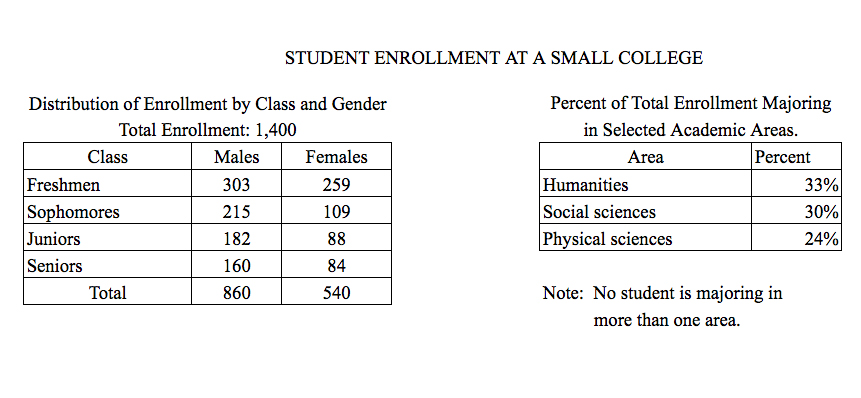# How many of the enrolled students are not majoring inHow many of the enrolled students are not majoring in humanities, social sciences, or physical sciences?

1. \$87\$
2. \$122\$
3. \$182\$
4. \$230\$
5. \$322\$

So, you were trying to be a good test taker and practice for the GRE with PowerPrep online. Buuuut then you had some questions about the quant section—specifically question 15 of Section 6 of Practice Test 1. Those questions testing our knowledge of Graphical Methods for Describing Data can be kind of tricky, but never fear, PrepScholar has got your back!

## Survey the Question

Let’s search the problem for clues as to what it will be testing, as this will help shift our minds to think about what type of math knowledge we’ll use to solve this question. Pay attention to any words that sound math-specific and anything special about what the numbers look like, and mark them on our paper.

Looks like we’ll need to analyze two tables containing data in this question, so it likely will draw on what we’ve learned about Graphical Methods for Describing Data. Let’s keep what we’ve learned about this skill at the tip of our minds as we approach this question.

## What Do We Know?

Let’s carefully read through the question and make a list of the things that we know.

1. We want to find the number of students not majoring in either of the three majors listed in the second table

## Develop a Plan

Let’s quickly scan the two tables to see what they have. The first table seems to be a two-way table for the class of a student (Freshmen, Sophomores, Juniors, or Seniors) and the gender of a student (Male or Female). The second table appears to show the percent of all students in three different majors.

Of course, we might have noticed that the second table looked incomplete. There’s only three majors listed. So if it listed \$100%\$ of the students, then it should have, on average, about \$33%\$ for each entry. While one major is listed at \$33%\$, the others are less. There must be something missing here.

So in the end, this problem was actually kind of predictable. They left out a “None of these Three Majors” category, which we could also describe as “students…not majoring in humanities, social sciences, or physical sciences,” coincidentally.
It looks like the path to answering how many of these “None of these Three Majors” students there are:

1. Find out what percent of students are not majoring in either of these three majors
2. Use that percent to multiply with the number of students to convert to a number of students

So let’s get to it!

## Solve the Question

First, we know that all of the students comprise \$100%\$ of the students. So if we subtract the three percentages listed for the majors in the second table from \$100%\$, we’ll have the percent of students not majoring in one of these three majors.

\$\$\Percent \Majoring \Neither = 100% – 33% – 30% – 24%\$\$

Using our calculator here, we see that we’ll get \$13%\$ for the percent of students who are not majoring in either humanities, social sciences, or physical sciences.

Whew! Halfway done with this question. Now, we do need the number of students, not just the percentage. The first table tells us that there are \$1{,}400\$ students. So let’s multiply the percentage we just found by the total number of students to get the number of students not majoring in either humanities, social sciences, or physical sciences. Of course, we’ll remember to divide our percentage by \$100\$ before using it for a calculation, like we always should.

\$\$\Number \Majoring \Neither = 13/100·1{,}400\$\$

Using our calculator here, we can see that there are \$182\$ students not majoring in humanities, social sciences, or physical sciences. The correct answer is C, \$182\$.

## What Did We Learn

Always think critically about the numbers we see when it comes to Data questions displayed with tables and graphs. Here,
we could almost predict that a question would focus on the missing major percentage, as we’re used to seeing percents add up to \$100\$.

Want more expert GRE prep? Sign up for the five-day free trial of our PrepScholar GRE Online Prep Program to access your personalized study plan with 90 interactive lessons and over 1600 GRE questions.